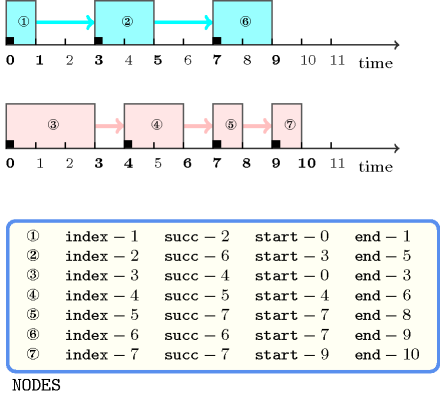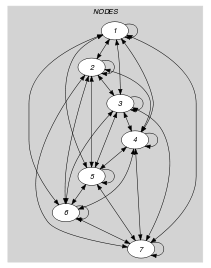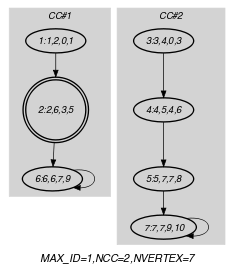## 5.400. temporal_path

Origin

ILOG

Constraint

$\mathrm{𝚝𝚎𝚖𝚙𝚘𝚛𝚊𝚕}_\mathrm{𝚙𝚊𝚝𝚑}\left(\mathrm{𝙽𝙿𝙰𝚃𝙷},\mathrm{𝙽𝙾𝙳𝙴𝚂}\right)$

Arguments
 $\mathrm{𝙽𝙿𝙰𝚃𝙷}$ $\mathrm{𝚍𝚟𝚊𝚛}$ $\mathrm{𝙽𝙾𝙳𝙴𝚂}$ $\mathrm{𝚌𝚘𝚕𝚕𝚎𝚌𝚝𝚒𝚘𝚗}\left(\begin{array}{c}\mathrm{𝚒𝚗𝚍𝚎𝚡}-\mathrm{𝚒𝚗𝚝},\hfill \\ \mathrm{𝚜𝚞𝚌𝚌}-\mathrm{𝚍𝚟𝚊𝚛},\hfill \\ \mathrm{𝚜𝚝𝚊𝚛𝚝}-\mathrm{𝚍𝚟𝚊𝚛},\hfill \\ \mathrm{𝚎𝚗𝚍}-\mathrm{𝚍𝚟𝚊𝚛}\hfill \end{array}\right)$
Restrictions
 $\mathrm{𝙽𝙿𝙰𝚃𝙷}\ge 1$ $\mathrm{𝙽𝙿𝙰𝚃𝙷}\le |\mathrm{𝙽𝙾𝙳𝙴𝚂}|$ $\mathrm{𝚛𝚎𝚚𝚞𝚒𝚛𝚎𝚍}$$\left(\mathrm{𝙽𝙾𝙳𝙴𝚂},\left[\mathrm{𝚒𝚗𝚍𝚎𝚡},\mathrm{𝚜𝚞𝚌𝚌},\mathrm{𝚜𝚝𝚊𝚛𝚝},\mathrm{𝚎𝚗𝚍}\right]\right)$ $|\mathrm{𝙽𝙾𝙳𝙴𝚂}|>0$ $\mathrm{𝙽𝙾𝙳𝙴𝚂}.\mathrm{𝚒𝚗𝚍𝚎𝚡}\ge 1$ $\mathrm{𝙽𝙾𝙳𝙴𝚂}.\mathrm{𝚒𝚗𝚍𝚎𝚡}\le |\mathrm{𝙽𝙾𝙳𝙴𝚂}|$ $\mathrm{𝚍𝚒𝚜𝚝𝚒𝚗𝚌𝚝}$$\left(\mathrm{𝙽𝙾𝙳𝙴𝚂},\mathrm{𝚒𝚗𝚍𝚎𝚡}\right)$ $\mathrm{𝙽𝙾𝙳𝙴𝚂}.\mathrm{𝚜𝚞𝚌𝚌}\ge 1$ $\mathrm{𝙽𝙾𝙳𝙴𝚂}.\mathrm{𝚜𝚞𝚌𝚌}\le |\mathrm{𝙽𝙾𝙳𝙴𝚂}|$ $\mathrm{𝙽𝙾𝙳𝙴𝚂}.\mathrm{𝚜𝚝𝚊𝚛𝚝}\le \mathrm{𝙽𝙾𝙳𝙴𝚂}.\mathrm{𝚎𝚗𝚍}$
Purpose

Let $G$ be the digraph described by the $\mathrm{𝙽𝙾𝙳𝙴𝚂}$ collection. Partition $G$ with a set of disjoint paths such that each vertex of the graph belongs to a single path. In addition, for all pairs of consecutive vertices of a path we have a precedence constraint that enforces the end associated with the first vertex to be less than or equal to the start related to the second vertex.

Example
$\left(\begin{array}{c}2,〈\begin{array}{cccc}\mathrm{𝚒𝚗𝚍𝚎𝚡}-1\hfill & \mathrm{𝚜𝚞𝚌𝚌}-2\hfill & \mathrm{𝚜𝚝𝚊𝚛𝚝}-0\hfill & \mathrm{𝚎𝚗𝚍}-1,\hfill \\ \mathrm{𝚒𝚗𝚍𝚎𝚡}-2\hfill & \mathrm{𝚜𝚞𝚌𝚌}-6\hfill & \mathrm{𝚜𝚝𝚊𝚛𝚝}-3\hfill & \mathrm{𝚎𝚗𝚍}-5,\hfill \\ \mathrm{𝚒𝚗𝚍𝚎𝚡}-3\hfill & \mathrm{𝚜𝚞𝚌𝚌}-4\hfill & \mathrm{𝚜𝚝𝚊𝚛𝚝}-0\hfill & \mathrm{𝚎𝚗𝚍}-3,\hfill \\ \mathrm{𝚒𝚗𝚍𝚎𝚡}-4\hfill & \mathrm{𝚜𝚞𝚌𝚌}-5\hfill & \mathrm{𝚜𝚝𝚊𝚛𝚝}-4\hfill & \mathrm{𝚎𝚗𝚍}-6,\hfill \\ \mathrm{𝚒𝚗𝚍𝚎𝚡}-5\hfill & \mathrm{𝚜𝚞𝚌𝚌}-7\hfill & \mathrm{𝚜𝚝𝚊𝚛𝚝}-7\hfill & \mathrm{𝚎𝚗𝚍}-8,\hfill \\ \mathrm{𝚒𝚗𝚍𝚎𝚡}-6\hfill & \mathrm{𝚜𝚞𝚌𝚌}-6\hfill & \mathrm{𝚜𝚝𝚊𝚛𝚝}-7\hfill & \mathrm{𝚎𝚗𝚍}-9,\hfill \\ \mathrm{𝚒𝚗𝚍𝚎𝚡}-7\hfill & \mathrm{𝚜𝚞𝚌𝚌}-7\hfill & \mathrm{𝚜𝚝𝚊𝚛𝚝}-9\hfill & \mathrm{𝚎𝚗𝚍}-10\hfill \end{array}〉\hfill \end{array}\right)$

The $\mathrm{𝚝𝚎𝚖𝚙𝚘𝚛𝚊𝚕}_\mathrm{𝚙𝚊𝚝𝚑}$ constraint holds since:

• The items of the $\mathrm{𝙽𝙾𝙳𝙴𝚂}$ collection represent the two ($\mathrm{𝙽𝙿𝙰𝚃𝙷}=2$) paths $1\to 2\to 6$ and $3\to 4\to 5\to 7$.

• As illustrated by Figure 5.400.1, all precedences between adjacent vertices of a same path hold: each item $i$ ($1\le i\le 7$) of the $\mathrm{𝙽𝙾𝙳𝙴𝚂}$ collection is represented by a rectangle starting and ending at instants $\mathrm{𝙽𝙾𝙳𝙴𝚂}\left[i\right].\mathrm{𝚜𝚝𝚊𝚛𝚝}$ and $\mathrm{𝙽𝙾𝙳𝙴𝚂}\left[i\right].\mathrm{𝚎𝚗𝚍}$; the number within each rectangle designates the index of the corresponding item within the $\mathrm{𝙽𝙾𝙳𝙴𝚂}$ collection.

##### Figure 5.400.1. The two paths of the Example slot represented as two sequences of tasksTypical
 $\mathrm{𝙽𝙿𝙰𝚃𝙷}<|\mathrm{𝙽𝙾𝙳𝙴𝚂}|$ $|\mathrm{𝙽𝙾𝙳𝙴𝚂}|>1$ $\mathrm{𝙽𝙾𝙳𝙴𝚂}.\mathrm{𝚜𝚝𝚊𝚛𝚝}<\mathrm{𝙽𝙾𝙳𝙴𝚂}.\mathrm{𝚎𝚗𝚍}$
Symmetries
• Items of $\mathrm{𝙽𝙾𝙳𝙴𝚂}$ are permutable.

• One and the same constant can be added to the $\mathrm{𝚜𝚝𝚊𝚛𝚝}$ and $\mathrm{𝚎𝚗𝚍}$ attributes of all items of $\mathrm{𝙽𝙾𝙳𝙴𝚂}$.

Arg. properties

Functional dependency: $\mathrm{𝙽𝙿𝙰𝚃𝙷}$ determined by $\mathrm{𝙽𝙾𝙳𝙴𝚂}$.

Remark

This constraint is related to the $\mathrm{𝚙𝚊𝚝𝚑}$ constraint of Ilog Solver. It can also be directly expressed with the $\mathrm{𝚌𝚢𝚌𝚕𝚎}$ [BeldiceanuContejean94] constraint of CHIP by using the diff nodes and the origin parameters. A generic model based on linear programming that handles paths, trees and cycles is presented in [LabbeLaporteRodriguezMartin98].

Reformulation

The $\mathrm{𝚝𝚎𝚖𝚙𝚘𝚛𝚊𝚕}_\mathrm{𝚙𝚊𝚝𝚑}$$\left(\mathrm{𝙽𝙿𝙰𝚃𝙷},\mathrm{𝙽𝙾𝙳𝙴𝚂}\right)$ constraint can be expressed in term of a conjunction of one $\mathrm{𝚙𝚊𝚝𝚑}$ constraint, $|\mathrm{𝙽𝙾𝙳𝙴𝚂}|$ $\mathrm{𝚎𝚕𝚎𝚖𝚎𝚗𝚝}$ constraints, and $|\mathrm{𝙽𝙾𝙳𝙴𝚂}|$ inequalities constraints:

• We pass to the $\mathrm{𝚙𝚊𝚝𝚑}$ constraint the number of path variable $\mathrm{𝙽𝙿𝙰𝚃𝙷}$ as well as the items of the $\mathrm{𝙽𝙾𝙳𝙴𝚂}$ collection form which we remove the $\mathrm{𝚜𝚝𝚊𝚛𝚝}$ and $\mathrm{𝚎𝚗𝚍}$ attributes.

• To the $i$-th $\left(1\le i\le |\mathrm{𝙽𝙾𝙳𝙴𝚂}|\right)$ item of the $\mathrm{𝙽𝙾𝙳𝙴𝚂}$ collection, we create a variable ${\mathrm{𝑆𝑡𝑎𝑟𝑡}}_{{\mathrm{𝑠𝑢𝑐𝑐}}_{𝑖}}$ and an $\mathrm{𝚎𝚕𝚎𝚖𝚎𝚗𝚝}$$\left(\mathrm{𝙽𝙾𝙳𝙴𝚂}\left[i\right].\mathrm{𝚜𝚞𝚌𝚌},〈{T}_{i,1},{T}_{i,2},\cdots ,{T}_{i,\mathrm{𝙽𝙾𝙳𝙴𝚂}}〉,{\mathrm{𝑆𝑡𝑎𝑟𝑡}}_{{\mathrm{𝑠𝑢𝑐𝑐}}_{𝑖}}\right)$ constraint, where ${T}_{i,j}=\mathrm{𝙽𝙾𝙳𝙴𝚂}\left[i\right].\mathrm{𝚜𝚝𝚊𝚛𝚝}$ if $i\ne j$ and ${T}_{i,i}=\mathrm{𝙽𝙾𝙳𝙴𝚂}\left[i\right].\mathrm{𝚎𝚗𝚍}$ otherwise.

• Finaly to the $i$-th $\left(1\le i\le |\mathrm{𝙽𝙾𝙳𝙴𝚂}|\right)$ item of the $\mathrm{𝙽𝙾𝙳𝙴𝚂}$ collection, we also create an inequality constraint $\mathrm{𝙽𝙾𝙳𝙴𝚂}\left[i\right].\mathrm{𝚎𝚗𝚍}\le {\mathrm{𝑆𝑡𝑎𝑟𝑡}}_{{\mathrm{𝑠𝑢𝑐𝑐}}_{𝑖}}$. Note that, since ${T}_{i,i}$ was initialised to $\mathrm{𝙽𝙾𝙳𝙴𝚂}\left[i\right].\mathrm{𝚎𝚗𝚍}$, the inequality $\mathrm{𝙽𝙾𝙳𝙴𝚂}\left[i\right].\mathrm{𝚎𝚗𝚍}\le {T}_{i,j}$ holds when $i=j$.

With respect to the Example slot we get the following conjunction of constraints:

$\mathrm{𝚙𝚊𝚝𝚑}$$\left(2,〈\mathrm{𝚒𝚗𝚍𝚎𝚡}-1\mathrm{𝚜𝚞𝚌𝚌}-2,\mathrm{𝚒𝚗𝚍𝚎𝚡}-2\mathrm{𝚜𝚞𝚌𝚌}-6,\mathrm{𝚒𝚗𝚍𝚎𝚡}-3\mathrm{𝚜𝚞𝚌𝚌}-4,$

$\mathrm{𝚒𝚗𝚍𝚎𝚡}-4\mathrm{𝚜𝚞𝚌𝚌}-5,\mathrm{𝚒𝚗𝚍𝚎𝚡}-5\mathrm{𝚜𝚞𝚌𝚌}-7,\mathrm{𝚒𝚗𝚍𝚎𝚡}-6\mathrm{𝚜𝚞𝚌𝚌}-6,$

$\mathrm{𝚒𝚗𝚍𝚎𝚡}-7\mathrm{𝚜𝚞𝚌𝚌}-7〉\right)$,

$\mathrm{𝚎𝚕𝚎𝚖𝚎𝚗𝚝}$$\left(2,〈1,3,0,4,7,7,9〉,3\right)$,

$\mathrm{𝚎𝚕𝚎𝚖𝚎𝚗𝚝}$$\left(6,〈1,5,0,4,7,7,9〉,7\right)$,

$\mathrm{𝚎𝚕𝚎𝚖𝚎𝚗𝚝}$$\left(4,〈1,5,3,4,7,7,9〉,4\right)$,

$\mathrm{𝚎𝚕𝚎𝚖𝚎𝚗𝚝}$$\left(5,〈1,5,3,6,7,7,9〉,7\right)$,

$\mathrm{𝚎𝚕𝚎𝚖𝚎𝚗𝚝}$$\left(7,〈1,5,3,6,8,7,9〉,9\right)$,

$\mathrm{𝚎𝚕𝚎𝚖𝚎𝚗𝚝}$$\left(6,〈1,5,3,6,8,9,9〉,9\right)$,

$\mathrm{𝚎𝚕𝚎𝚖𝚎𝚗𝚝}$$\left(7,〈1,5,3,6,8,9,10〉,10\right)$,

$1\le 3$, $5\le 7$, $3\le 4$, $6\le 7$, $8\le 9$, $9\le 9$, $10\le 10$.

specialisation: $\mathrm{𝚙𝚊𝚝𝚑}$ (time dimension removed).

Keywords
Arc input(s)

$\mathrm{𝙽𝙾𝙳𝙴𝚂}$

Arc generator
$\mathrm{𝐶𝐿𝐼𝑄𝑈𝐸}$$↦\mathrm{𝚌𝚘𝚕𝚕𝚎𝚌𝚝𝚒𝚘𝚗}\left(\mathrm{𝚗𝚘𝚍𝚎𝚜}\mathtt{1},\mathrm{𝚗𝚘𝚍𝚎𝚜}\mathtt{2}\right)$

Arc arity
Arc constraint(s)
 $•\mathrm{𝚗𝚘𝚍𝚎𝚜}\mathtt{1}.\mathrm{𝚜𝚞𝚌𝚌}=\mathrm{𝚗𝚘𝚍𝚎𝚜}\mathtt{2}.\mathrm{𝚒𝚗𝚍𝚎𝚡}$ $•\mathrm{𝚗𝚘𝚍𝚎𝚜}\mathtt{1}.\mathrm{𝚜𝚞𝚌𝚌}=\mathrm{𝚗𝚘𝚍𝚎𝚜}\mathtt{1}.\mathrm{𝚒𝚗𝚍𝚎𝚡}\vee \mathrm{𝚗𝚘𝚍𝚎𝚜}\mathtt{1}.\mathrm{𝚎𝚗𝚍}\le \mathrm{𝚗𝚘𝚍𝚎𝚜}\mathtt{2}.\mathrm{𝚜𝚝𝚊𝚛𝚝}$ $•\mathrm{𝚗𝚘𝚍𝚎𝚜}\mathtt{1}.\mathrm{𝚜𝚝𝚊𝚛𝚝}\le \mathrm{𝚗𝚘𝚍𝚎𝚜}\mathtt{1}.\mathrm{𝚎𝚗𝚍}$ $•\mathrm{𝚗𝚘𝚍𝚎𝚜}\mathtt{2}.\mathrm{𝚜𝚝𝚊𝚛𝚝}\le \mathrm{𝚗𝚘𝚍𝚎𝚜}\mathtt{2}.\mathrm{𝚎𝚗𝚍}$
Graph property(ies)
 $•$$\mathrm{𝐌𝐀𝐗}_\mathrm{𝐈𝐃}$$\le 1$ $•$$\mathrm{𝐍𝐂𝐂}$$=\mathrm{𝙽𝙿𝙰𝚃𝙷}$ $•$$\mathrm{𝐍𝐕𝐄𝐑𝐓𝐄𝐗}$$=|\mathrm{𝙽𝙾𝙳𝙴𝚂}|$

Graph model

The arc constraint is a conjunction of four conditions that respectively correspond to:

• A constraint that links the successor variable of a first vertex to the index attribute of a second vertex,

• A precedence constraint that applies on one vertex and its distinct successor,

• One precedence constraint between the start and the end of the vertex that corresponds to the departure of an arc,

• One precedence constraint between the start and the end of the vertex that corresponds to the arrival of an arc.

We use the following three graph properties in order to enforce the partitioning of the graph in distinct paths:

Parts (A) and (B) of Figure 5.400.2 respectively show the initial and final graph associated with the Example slot. Since we use the $\mathrm{𝐌𝐀𝐗}_\mathrm{𝐈𝐃}$, the $\mathrm{𝐍𝐂𝐂}$ and the $\mathrm{𝐍𝐕𝐄𝐑𝐓𝐄𝐗}$ graph properties we display the following information on the final graph:

• We show with a double circle a vertex that has the maximum number of predecessors.

• We show the two connected components corresponding to the two paths.

• We put in bold the vertices.

##### Figure 5.400.2. Initial and final graph of the $\mathrm{𝚝𝚎𝚖𝚙𝚘𝚛𝚊𝚕}_\mathrm{𝚙𝚊𝚝𝚑}$ constraint(a) (b)